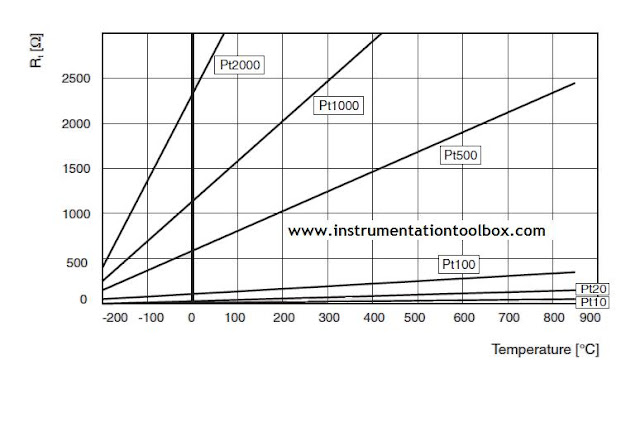The Callendar Van Dusen Equation for Platinum Based RTD Thermometers ~ Learning Instrumentation And Control Engineering Learning Instrumentation And Control Engineering

### The Callendar Van Dusen Equation for Platinum Based RTD Thermometers

Custom Search

The Callendar-Van-Dusen equation describes the relationship between the Ohmic Resistance and Temperature of Platinum based temperature instruments. This equation is commonly used in commercial applications in RTD thermometers and RTD Transmitters. In its original form, the Callendar-Van-Dusen Equation is given by:

Rt = R0 + R0α[t – δ(0.01t – 1)(0.01t) – β(0.01t – 1)(0.01t)3]

Where:
Rt = Resistance at Temperature t (°C)
R0  = Resistance at t = 0°C
α  = Sensor specific contant
δ  = Sensor specific constant
β  = Sensor specific constant (0 at t > 0°C, 0.11 at t < 0°C)

The above equation has been used in the EN/IEC 60751 standard in the format below:

Rt = R0(1 + At + Bt2)      for t ≥ 0°C,  for a Platinum resistor, t is in the range -200°C to 0°C
Rt = R0(1 + At + Bt2 + C(t - 100)t3  for  t ≤ 0°C,
for a Platinum resistor, t is in the range 0°C to 850°C

The value R0 is the resistance of the Platinum resistor at 0°C. The coefficients A, B, C as well as all other properties which the platinum resistance based temperature instrument must satisfy are contained in the EN/IEC 60751 standard.

Nominal Values of Platinum Used as Temperature Measurement Devices.
For industrial temperature measurement, Platinum is the most used resistors followed by Nickel. Platinum measurement resistors with a nominal value of 100 ohms known as Pt 100 have been established as the industrial standard for temperature measurement.

Temperature resistors are identified by their resistance at 0oC also called the nominal value. Pt 100 and Ni 100 the most common types of resistors used have a resistance of 100 ohms at 0oC. Pt 2000, Pt 1000, Pt 500, Pt 20, Pt 10 have 2000 ohms, 1000 ohms, 500 ohms, 20 ohms and 10 ohms respectively at 0°C. The relationship between resistance and temperature for various types of Platinum resistance is shown in the chart below:In the IEC/EN 60751 standard, the constants A, B & C are given the following values:

A = 3.9083 x 10-3 K-1

B = -5.775 x  10-7 K-2

C = -4.183 X 10-12 K-4

Application of the Callendar – Van – Dusen Equation
It is used in transmitter-sensor matching to create a curve that closely approximates an RTD’s resistance versus temperature relationship. This curve can be generated for any RTD by plugging the RTD’s specific constants (A, B & C) into either version of the Callendar-Van Dusen equation, which is programmed into many smart transmitters.  In this way, the transmitter uses the actual RTD curve rather than an ideal curve to translate the sensor’s resistance signal into a temperature value thus providing extraordinary system accuracy.

Although transmitter-sensor matching is typically not required for all process measurements, it is the clear choice for those measurements requiring the best possible accuracy.